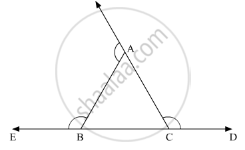Advertisement Remove all ads

# If the Sides of a Triangle Are Produced in Order, Then the Sum of the Three Exterior Angles So Formed is - Mathematics

MCQ

If the sides of a triangle are produced in order, then the sum of the three exterior angles so formed is

#### Options

• 90°

• 180°

• 270°

• 360°

Advertisement Remove all ads

#### Solution

n the given ΔABC, all the three sides of the triangle are produced. We need to find the sum of the three exterior angles so produced.Now, according to the angle sum property of the triangle

∠A + ∠B + ∠C = 180°         .......(1)

Further, using the property, “an exterior angle of the triangle is equal to the sum of two opposite interior angles”, we get,

ext.∠C = ∠A + ∠B           ......(2)

Similarly,

ext.∠A = ∠C + ∠B            .......(3)

Also,

ext.∠B = ∠C+ ∠A            .......(4)

Adding (2) (3) and (4)

We get,

ext.∠A + ext.∠B+ ext.∠C = ∠C+∠B +∠C +∠A + ∠B + ∠A

= 2(∠A= ∠B+ ∠C   )

= 2 (180°)

= 360°

Thus,   ext. ∠A + ext. ∠B ext.∠C  = 360°

Is there an error in this question or solution?
Advertisement Remove all ads

#### APPEARS IN

RD Sharma Mathematics for Class 9
Chapter 11 Triangle and its Angles
Q 8 | Page 25
Advertisement Remove all ads
Advertisement Remove all ads
Share
Notifications

View all notifications

Forgot password?
Course